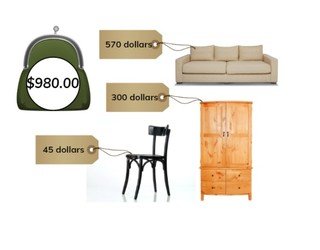Subtraction to 1,000 with three or more numbers

# Subtraction to 1,000 with three or more numbers

No account needed.8,000 schools use Gynzy92,000 teachers use Gynzy1,600,000 students use Gynzy

## General

Students learn to subtract numbers to 1,000 with three or more numbers.

2.NBT.B.7

## Relevance

It is important to be able to subtract numbers to 1,000 with multiple numbers so you can determine how much you have left when multiple numbers are taken away. An example would be if you saved money and now bought a new tv and a new radio and want to determine how much money you have left.

## Introduction

Practice the number to 1,000 with students by dragging the MAB blocks on the interactive whiteboard. Then make a subtraction problem in which you drag blocks away to the box.

## Development

First discuss the importance of subtracting numbers to 1,000 with three or more numbers. Next, explain how you solve a subtraction problem with three or more numbers by using the example of placing numbers of blocks into a box. Explain to students that they can swap the order of the second, third, etc numbers, as long as they are all taken away from the first (or largest total) number. Students can choose the order in which to subtract that is easiest for them. The learning goal is presented in three ways, visually, in the abstract, and as story problems. Please use the navigation on the bottom right to select the explanation that is best suited to your classroom.
In the visual form, the subtraction problem is shown by MAB blocks on the interactive whiteboard. There is extra emphasis on the fact that you can swap the second/third (etc) subtrahends to make the subtraction problem easier to solve. In the abstract, students are given the numbers without visual support. You can tell students to imagine the subtraction problem only having one subtrahend, and to use that difference to subtract the second number (subtrahend) from. Show students that you can also choose to add the second and third subtrahends together to take that away from the first number (minuend) in one go. Show students how to solve a subtraction problem shown as a table- in which the blanks equal the numbers that together total the number given at the top. Remind students how to solve a story problem and solve the first as a class, and ask the students to solve the next two individually or in pairs.

To check that students are able to subtract numbers to 1,000 with three or more numbers, you can ask the following questions:
- Why is it useful to be able to subtract three or more numbers?
- Why can it be useful to swap subtrahends- or to change the order of the subtrahends?

## Guided practice

Students are given subtraction problems with visual support, in the abstract and as story problems.

## Closing

Remind the students that it is important to be able to subtract by three or more numbers. Then divide the class into three groups. Each student in the first group writes a number between 800 and 1,000. Each student in the other two groups writes down a number between 0 and 400. Then students must form smaller groups of 3 (or more) with one member of each group. They then solve the subtraction problem their numbers create. You can repeat and change groups, or simply ask new groups to form using the same numbers.

## Teaching tips

Students who have difficulty with this learning goal can make use of blocks or other manipulatives to help visualize the process. If you create the piles of each number below each other, you can physically show how you take them away.

## Instruction materials

Optional: Blocks or other manipulatives

### The online teaching platform for interactive whiteboards and displays in schools

• Save time building lessons

• Manage the classroom more efficiently

• Increase student engagement